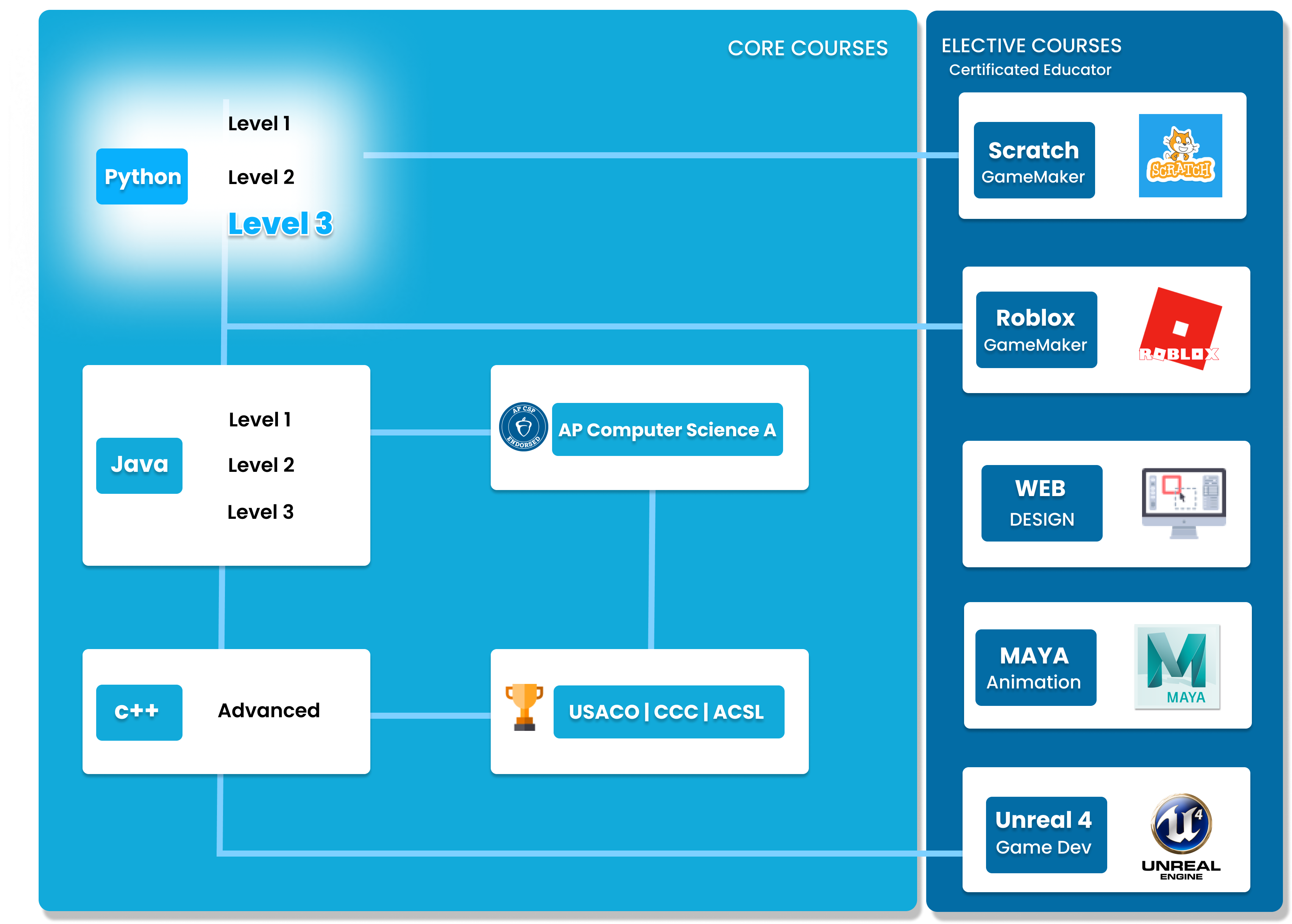# Python Level 3

Once students have fully understood all the fundamentals of Python, students will learn how to apply the concepts in order to create practical projects, which they can showcase on their portfolio. The projects created are done by creating applications, using external libraries. The topics covered in this level are: Game Development, Data Analyzing, build and use AI. We provide a variety of project topics, like “Detect text in images”, “wikipedia web scraping”...etc.##### Who is this course for
Beginner Students: This course is for students who have completed both Level 1 and 2 or have previous experience in coding.
This course typically takes 3-6 months to complete. This depends on the student's experience and how fast they can master the concepts and the knowledge. Our instructors move at the pace of the student, it may take extra time for some students to reinforce what they have learned.
##### Curriculum
###### Chapter 1: Introduction to AIChapter 1 introduces AI, what it is, and how it is programmed. Students will learn about the different branches of AI and Machine Learning techniques.
###### Chapter 2: Reviewing Levels 1 and 2Before creating complex projects and working with libraries, students will revise all the concepts from Levels 1 and 2 in order to check and solidify their understanding of the basics of Python.
###### Chapter 3: GUIsChapter 3 focuses on using the built-in Tkinter library, in order to create interactive GUIs. Students will recreate their previous projects and convert it into a GUI application.
###### Chapter 4: File I/OChapter 4 focuses on File input and output, and how to work with .txt and .csv files with Python.
###### Chapter 5: Google Cloud VisionChapter 5 uses the Google Cloud Vision API in order to create an OCR program. Students will learn how to program an AI to analyze images and identify objects.
###### Chapter 6: Web ScrapingIn this level, students will learn how to scrape the web using BeautifulSoup.
###### Chapter 7: Data VisualizationStudents will learn how to use Python with data science, and data visualization. Using the Matplotlib library, students can create graphs and charts from tables or data structures.
###### Chapter 8: Capstone ProjectChoose your favourite project topic. Complete with all knowledge that you learned from Python Level 1, 2, and 3.

### You Might Also Be Interested In Our Elective Courses##### Introduction to Number Theory
AGES 10 - 15

This course is designed to build the basics of a complicated mathematical field. It is designed to be a complete introduction to the fundamental concepts of number theory for students in grades 7-10.

The class covers topics such as primes and composites; multiples, divisors, and divisibility; prime factorization and its uses; modular arithmetic and more.##### Introduction to Geometry
AGES 10 - 15

Students in grades 6-10 will build a solid understanding of more advanced geometry concepts in this course. It is designed to expand on the standard high school geometry curriculum and to challenge students with new concepts and problems.

Topics include congruent and similar triangles, circles, 3D geometry, and introductory trigonometry.##### Introduction to Counting and Probability
AGES 10 - 15

To build on the concepts from the Introduction level course, students will build on their Counting and Probability knowledge.

A thorough introduction for students in grades 7-10 to counting and probability topics such as permutations, combinations, Pascal's triangle, geometric probability, basic combinatorial identities, the Binomial Theorem, Pigeonhole Principle Conditional, Probability Graph Theory and more.